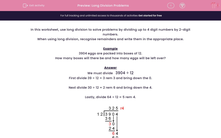# Long Division Problems

In this worksheet, students must solve problems involving dividing numbers up to 4 digits by a two-digit number using the formal written method of long division. There will be remainders, which should be interpreted correctly.This content is premium and exclusive to EdPlace subscribers.Key stage:  KS 2

Curriculum topic:   Maths and Numerical Reasoning

Curriculum subtopic:   Word Problems

Difficulty level:#### Worksheet Overview

In this worksheet, use long division to solve problems by dividing up to 4 digit numbers by 2-digit numbers.

When using long division, recognise remainders and write them in the appropriate place.

Example

3904 eggs are packed into boxes of 12.

How many boxes will there be and how many eggs will be left over?

We must divide   3904 ÷ 12

First divide 39 ÷ 12 = 3 rem 3 and bring down the 0.

Next divide 30 ÷ 12 = 2 rem 6 and bring down the 4.

Lastly, divide 64 ÷ 12 = 5 rem 4.We have shown that    3904 ÷ 12 = 325 r4

There will be 325 boxes and 4 eggs will be left over.

### What is EdPlace?

We're your National Curriculum aligned online education content provider helping each child succeed in English, maths and science from year 1 to GCSE. With an EdPlace account you’ll be able to track and measure progress, helping each child achieve their best. We build confidence and attainment by personalising each child’s learning at a level that suits them.

Get started